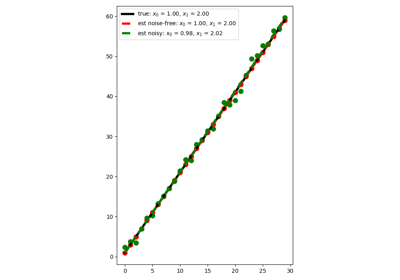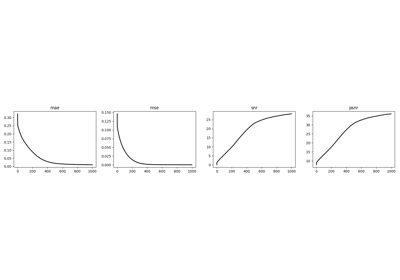# pylops.optimization.callback.Callbacks#

class pylops.optimization.callback.Callbacks[source]#

This is a template class which a user must subclass when implementing callbacks for a solver. This class comprises of the following methods:

• `on_setup_begin`: a method that is invoked at the start of the setup method of the solver

• `on_setup_end`: a method that is invoked at the end of the setup method of the solver

• `on_step_begin`: a method that is invoked at the start of the step method of the solver

• `on_step_end`: a method that is invoked at the end of the setup step of the solver

• `on_run_begin`: a method that is invoked at the start of the run method of the solver

• `on_run_end`: a method that is invoked at the end of the run method of the solver

All methods take two input parameters: the solver itself, and the vector `x`.

Examples

```>>> import numpy as np
>>> from pylops.basicoperators import MatrixMult
>>> from pylops.optimization.solver import CG
>>> from pylops.optimization.callback import Callbacks
>>> class StoreIterCallback(Callbacks):
...     def __init__(self):
...         self.stored = []
...     def on_step_end(self, solver, x):
...         self.stored.append(solver.iiter)
>>> cb_sto = StoreIterCallback()
>>> Aop = MatrixMult(np.random.normal(0., 1., 36).reshape(6, 6))
>>> Aop = Aop.H @ Aop
>>> y = Aop @ np.ones(6)
>>> cgsolve = CG(Aop, callbacks=[cb_sto, ])
>>> xest = cgsolve.solve(y=y, x0=np.zeros(6), tol=0, niter=6, show=False)
>>> xest
array([1., 1., 1., 1., 1., 1.])
```

Methods

 `__init__`() `on_run_begin`(solver, x) Callback before entire solver run `on_run_end`(solver, x) Callback after entire solver run `on_setup_begin`(solver, x0) Callback before setup `on_setup_end`(solver, x) Callback after setup `on_step_begin`(solver, x) Callback before step of solver `on_step_end`(solver, x) Callback after step of solver

## Examples using `pylops.optimization.callback.Callbacks`#Linear Regression

Linear Regression